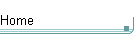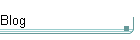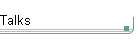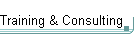GotW #38On theblogNovember 4: Other Concurrency Sessions at PDC November 3 October 26: Hoare on Testing October 23This is the original GotW problem and solution substantially as posted to Usenet. See the book More Exceptional C++ (Addison-Wesley, 2002) for the most current solution to this GotW issue. The solutions in the book have been revised and expanded since their initial appearance in GotW. The book versions also incorporate corrections, new material, and conformance to the final ANSI/ISO C++ standard.

## Multiple Inheritance - Part IIDifficulty: 8 / 10

If you couldn't use multiple inheritance, how would you emulate it? Don't forget to emulate as natural a syntax as possible for the client code.### Problem

1. Consider the following example:

```  struct A      { virtual ~A() { }
virtual string Name() { return "A";  } };
struct B1 : virtual A { string Name() { return "B1"; } };
struct B2 : virtual A { string Name() { return "B2"; } };

struct D  : B1, B2    { string Name() { return "D";  } };```

Demonstrate the best way you can find to "work around" not using multiple inheritance by writing an equivalent (or as near as possible) class D without using MI. How would you get the same effect and usability for D with as little change as possible to syntax in the client code?

Starter: You can begin by considering the cases in the following test harness.

```void f1( A&  x ) { cout << "f1:" << x.Name() << endl; }
void f2( B1& x ) { cout << "f2:" << x.Name() << endl; }
void f3( B2& x ) { cout << "f3:" << x.Name() << endl; }

void g1( A   x ) { cout << "g1:" << x.Name() << endl; }
void g2( B1  x ) { cout << "g2:" << x.Name() << endl; }
void g3( B2  x ) { cout << "g3:" << x.Name() << endl; }

int main() {
D   d;
B1* pb1 = &d;   // D* -> B* conversion
B2* pb2 = &d;
B1& rb1 = d;    // D& -> B& conversion
B2& rb2 = d;

f1( d );        // polymorphism
f2( d );
f3( d );

g1( d );        // slicing
g2( d );
g3( d );
// dynamic_cast/RTTI
cout << ( (dynamic_cast<D*>(pb1) != 0)
? "ok " : "bad " );
cout << ( (dynamic_cast<D*>(pb2) != 0)
? "ok " : "bad " );

try {
dynamic_cast<D&>(rb1);
cout << "ok ";
} catch(...) {
cout << "bad ";
}
try {
dynamic_cast<D&>(rb2);
cout << "ok ";
} catch(...) {
cout << "bad ";
}
}```### Solution

1. Consider the following example:

```  struct A      { virtual ~A() { }
virtual string Name() { return "A";  } };
struct B1 : virtual A { string Name() { return "B1"; } };
struct B2 : virtual A { string Name() { return "B2"; } };

struct D  : B1, B2    { string Name() { return "D";  } };```

Demonstrate the best way you can find to "work around" not using multiple inheritance by writing an equivalent (or as near as possible) class D without using MI. How would you get the same effect and usability for D with as little change as possible to syntax in the client code?

There are a few strategies, each with their weaknesses, but here's one that gets quite close:

```    struct D : B1 {
struct D2 : B2 {
void   Set ( D* d ) { d_ = d; }
string Name();
D* d_;
} d2_;

D()                 { d2_.Set( this ); }

D( const D& other ) : B1( other ), d2_( other.d2_ )
{ d2_.Set( this ); }

D& operator=( const D& other ) {
B1::operator=( other );
d2_ = other.d2_;
return *this;
}

operator B2&()      { return d2_; }

B2& AsB2()          { return d2_; }

string Name()       { return "D"; }
};
string D::D2::Name()    { return d_->Name(); }```

Some drawbacks are that:

- providing operator B2& arguably gives references special (inconsistent) treatment over pointers

- you need to call D::AsB2() explicitly to use a D as a B2 (in the test harness, this means changing "B2* pb2 = &d;" to "B2* pb2 = &d.AsB2();")

- dynamic_cast from D* to B2* still doesn't work (it's possible to work around this if you're willing to use the preprocessor to redefine dynamic_cast calls)

Interestingly, you may have observed that the D object layout in memory is probably identical to what multiple inheritance would give. That's because we're trying to simulate MI, just without all of the syntactic sugar and convenience that builtin language support would provide.